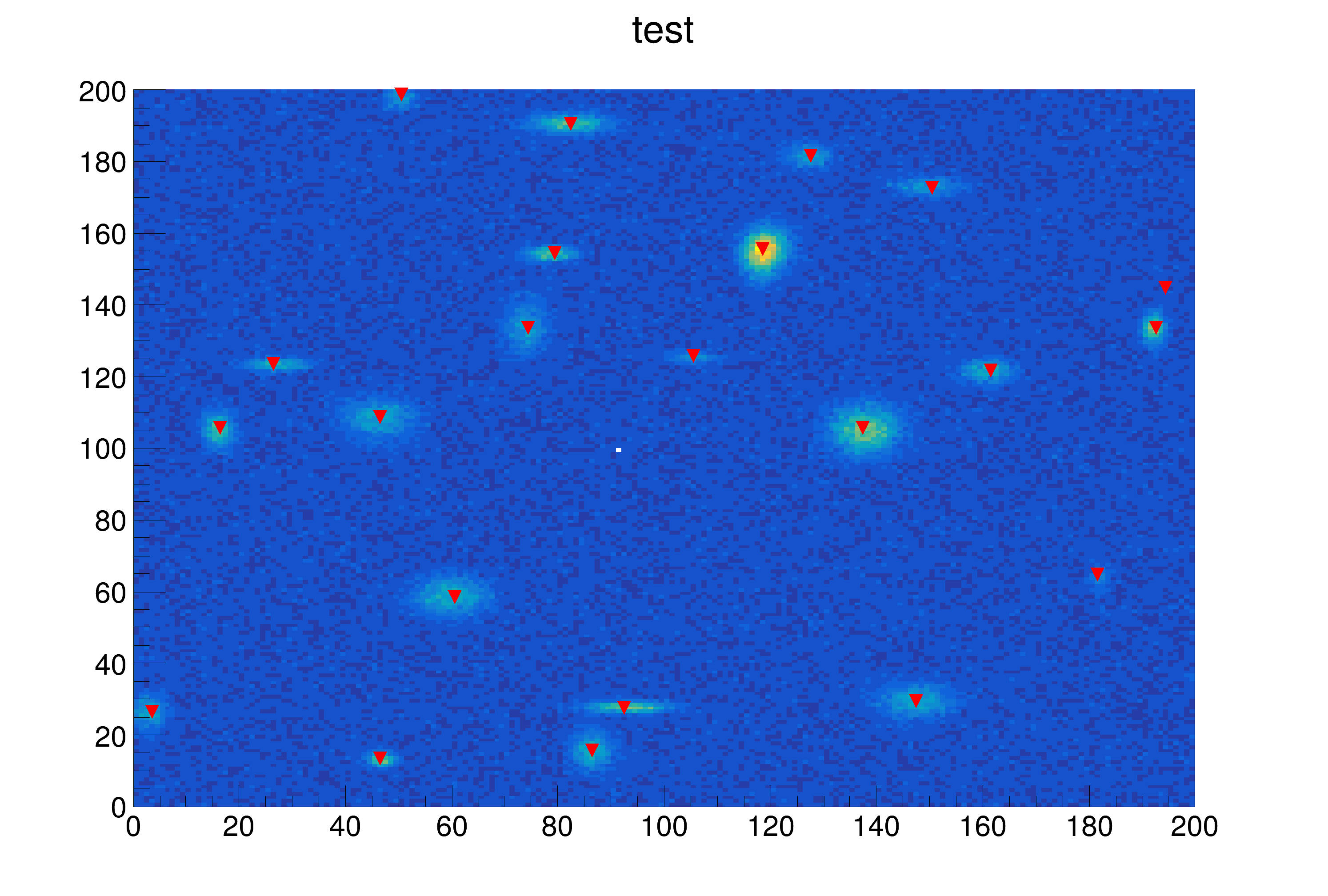ROOT   Reference Guide
Searching...
No Matches
peaks2.C File Reference

## Detailed DescriptionExample to illustrate the 2-d peak finder (class TSpectrum2).

This script generates a random number of 2-d gaussian peaks The position of the peaks is found via TSpectrum2 To execute this example, do:

root > .x peaks2.C (generate up to 50 peaks by default)
root > .x peaks2.C(10) (generate up to 10 peaks)
root > .x peaks2.C+(200) (generate up to 200 peaks via ACLIC)
Double_t x[n]
Definition legend1.C:17

The script will iterate generating a new histogram having between 5 and the maximun number of peaks specified. Double Click on the bottom right corner of the pad to go to a new spectrum To Quit, select the "quit" item in the canvas "File" menu#include "TSpectrum2.h"
#include "TCanvas.h"
#include "TRandom.h"
#include "TH2.h"
#include "TF2.h"
#include "TMath.h"
#include "TROOT.h"
TH2F *h2 = 0;
Int_t npeaks = 30;
Double_t fpeaks2(Double_t *x, Double_t *par) {
for (Int_t p=0;p<npeaks;p++) {
Double_t norm = par[5*p+0];
Double_t mean1 = par[5*p+1];
Double_t sigma1 = par[5*p+2];
Double_t mean2 = par[5*p+3];
Double_t sigma2 = par[5*p+4];
result += norm*TMath::Gaus(x,mean1,sigma1)*TMath::Gaus(x,mean2,sigma2);
}
return result;
}
void findPeak2() {
printf("Generating histogram with %d peaks\n",npeaks);
Int_t nbinsx = 200;
Int_t nbinsy = 200;
Double_t xmax = (Double_t)nbinsx;
Double_t ymax = (Double_t)nbinsy;
Double_t dx = (xmax-xmin)/nbinsx;
Double_t dy = (ymax-ymin)/nbinsy;
delete h2;
h2 = new TH2F("h2","test",nbinsx,xmin,xmax,nbinsy,ymin,ymax);
h2->SetStats(0);
//generate n peaks at random
Double_t par;
for (p=0;p<npeaks;p++) {
par[5*p+0] = gRandom->Uniform(0.2,1);
par[5*p+1] = gRandom->Uniform(xmin,xmax);
par[5*p+2] = gRandom->Uniform(dx,5*dx);
par[5*p+3] = gRandom->Uniform(ymin,ymax);
par[5*p+4] = gRandom->Uniform(dy,5*dy);
}
TF2 *f2 = new TF2("f2",fpeaks2,xmin,xmax,ymin,ymax,5*npeaks);
f2->SetNpx(100);
f2->SetNpy(100);
f2->SetParameters(par);
TCanvas *c1 = (TCanvas*)gROOT->GetListOfCanvases()->FindObject("c1");
if (!c1) c1 = new TCanvas("c1","c1",10,10,1000,700);
h2->FillRandom("f2",500000);
//now the real stuff: Finding the peaks
Int_t nfound = s->Search(h2,2,"col");
//searching good and ghost peaks (approximation)
Int_t pf,ngood = 0;
Double_t *xpeaks = s->GetPositionX();
Double_t *ypeaks = s->GetPositionY();
for (p=0;p<npeaks;p++) {
for (pf=0;pf<nfound;pf++) {
Double_t diffx = TMath::Abs(xpeaks[pf] - par[5*p+1]);
Double_t diffy = TMath::Abs(ypeaks[pf] - par[5*p+3]);
if (diffx < 2*dx && diffy < 2*dy) ngood++;
}
}
if (ngood > nfound) ngood = nfound;
//Search ghost peaks (approximation)
Int_t nghost = 0;
for (pf=0;pf<nfound;pf++) {
Int_t nf=0;
for (p=0;p<npeaks;p++) {
Double_t diffx = TMath::Abs(xpeaks[pf] - par[5*p+1]);
Double_t diffy = TMath::Abs(ypeaks[pf] - par[5*p+3]);
if (diffx < 2*dx && diffy < 2*dy) nf++;
}
if (nf == 0) nghost++;
}
c1->Update();
s->Print();
printf("Gener=%d, Found=%d, Good=%d, Ghost=%d\n",npeaks,nfound,ngood,nghost);
if (!gROOT->IsBatch()) {
printf("\nDouble click in the bottom right corner of the pad to continue\n");
c1->WaitPrimitive();
}
}
void peaks2(Int_t maxpeaks=50) {
s = new TSpectrum2(2*maxpeaks);
for (int i=0; i<10; ++i) {
npeaks = (Int_t)gRandom->Uniform(5,maxpeaks);
findPeak2();
}
}
int Int_t
Definition RtypesCore.h:45
double Double_t
Definition RtypesCore.h:59
winID h TVirtualViewer3D TVirtualGLPainter p
Option_t Option_t TPoint TPoint const char GetTextMagnitude GetFillStyle GetLineColor GetLineWidth GetMarkerStyle GetTextAlign GetTextColor GetTextSize void char Point_t Rectangle_t WindowAttributes_t Float_t Float_t Float_t Int_t Int_t UInt_t UInt_t Rectangle_t result
float xmin
float ymin
float xmax
float ymax
#define gROOT
Definition TROOT.h:406
R__EXTERN TRandom * gRandom
Definition TRandom.h:62
The Canvas class.
Definition TCanvas.h:23
virtual void SetNpx(Int_t npx=100)
Set the number of points used to draw the function.
Definition TF1.cxx:3431
virtual void SetParameters(const Double_t *params)
Definition TF1.h:649
A 2-Dim function with parameters.
Definition TF2.h:29
virtual void SetNpy(Int_t npy=100)
Set the number of points used to draw the function.
Definition TF2.cxx:928
virtual void SetStats(Bool_t stats=kTRUE)
Set statistics option on/off.
Definition TH1.cxx:8859
2-D histogram with a float per channel (see TH1 documentation)}
Definition TH2.h:258
void FillRandom(const char *fname, Int_t ntimes=5000, TRandom *rng=nullptr) override
Fill histogram following distribution in function fname.
Definition TH2.cxx:668
TObject * FindObject(const char *name) const override
virtual Double_t Uniform(Double_t x1=1)
Returns a uniform deviate on the interval (0, x1).
Definition TRandom.cxx:672
Definition TSpectrum2.h:18
Double_t * GetPositionY() const
Definition TSpectrum2.h:45
Double_t * GetPositionX() const
Definition TSpectrum2.h:44
void Print(Option_t *option="") const override
Print the array of positions.
virtual Int_t Search(const TH1 *hist, Double_t sigma=2, Option_t *option="", Double_t threshold=0.05)
This function searches for peaks in source spectrum in hin The number of found peaks and their positi...
return c1
Definition legend1.C:41
Double_t Gaus(Double_t x, Double_t mean=0, Double_t sigma=1, Bool_t norm=kFALSE)
Calculates a gaussian function with mean and sigma.
Definition TMath.cxx:471
Short_t Abs(Short_t d)
Returns the absolute value of parameter Short_t d.
Definition TMathBase.h:123

Definition in file peaks2.C.